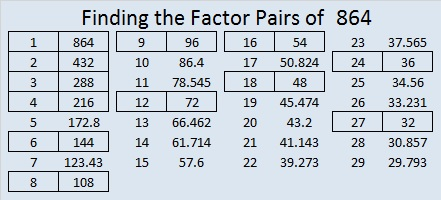# 864 Factor Trees

The prime factorization of 864 is 2⁵ × 3³. The sum of the exponents is 5 + 3 = 8. Since 8 is a power of 2,  a couple of 864’s factor trees are full and well-balanced:All of those prime factors lined up in numerical order. That didn’t happen for the next one, but it still makes a good looking tree, and all the prime factors are easy to find.Is it possible to make a factor tree for 864 that hardly looks like a tree and isn’t as easy to find all the prime factors? Yes, it is. Here’s an example:864 looked interesting to me in a few other bases:

• 4000 BASE 6 because 4(6³) = 864
• 600 BASE 12 because 6(12²) = 864
• RR BASE 31 (R is 27 base 10) because 27(31) + 27(1) = 27(32) = 864
• OO BASE 35 (O is 24 base 10) because 24(35) + 24(1) = 24(36) = 864
• O0 BASE 36 (Oh zero) because 24(36) + 0(1) = 864

864 is the sum of the 20 prime numbers from 7 to 83.

131 + 137 + 139 + 149 + 151 + 157 = 864; that’s six consecutive primes.

431 + 433 = 864; that’s the sum of twin primes.

• 864 is a composite number.
• Prime factorization: 864 = 2 × 2 × 2 × 2 × 2 × 3 × 3 × 3, which can be written 864 = 2⁵ × 3³
• The exponents in the prime factorization are 5 and 3. Adding one to each and multiplying we get (5 + 1)(3 + 1) = 6 × 4 = 24. Therefore 864 has exactly 24 factors.
• Factors of 864: 1, 2, 3, 4, 6, 8, 9, 12, 16, 18, 24, 27, 32, 36, 48, 54, 72, 96, 108, 144, 216, 288, 432, 864
• Factor pairs: 864 = 1 × 864, 2 × 432, 3 × 288, 4 × 216, 6 × 144, 8 × 108, 9 × 96, 12 × 72, 16 × 54, 18 × 48, 24 × 36, or 27 × 32
• Taking the factor pair with the largest square number factor, we get √864 = (√144)(√6) = 12√6 ≈ 29.3938769This site uses Akismet to reduce spam. Learn how your comment data is processed.